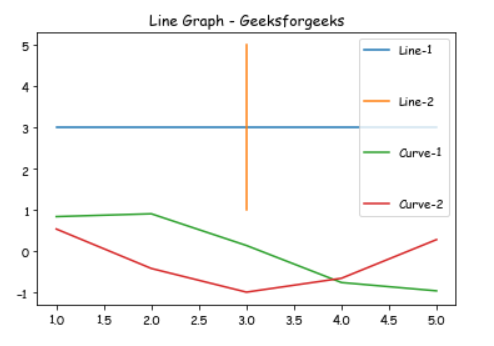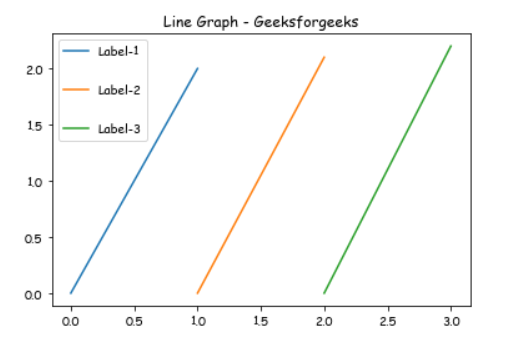Related Articles

# How Change the vertical spacing between legend entries in Matplotlib?

• Last Updated : 13 Jan, 2021

Prerequisites: Matplotlib

In this article, we will see how can we can change vertical space between labels of a legend in our graph using matplotlib, Here we will take two different examples to showcase our graph.

Approach:

• Import required module.
• Create data.
• Change the vertical spacing between labels.
• Normally plot the data.
• Display plot.

Implementation:

Example 1:

In this example, we will draw different lines with the help of matplotlib and Use the labelspacing argument to plt.legend() to change the vertical space between labels.

## Python3

 `# importing package ` `import` `matplotlib.pyplot as plt ` `import` `numpy as np ` ` `  `# create data ` `X ``=` `[``1``, ``2``, ``3``, ``4``, ``5``] ` `Y ``=` `[``3``, ``3``, ``3``, ``3``, ``3``] ` ` `  `# plot lines ` `plt.plot(X, Y, label ``=` `"Line-1"``) ` `plt.plot(Y, X, label ``=` `"Line-2"``) ` `plt.plot(X, np.sin(X), label ``=` `"Curve-1"``) ` `plt.plot(X, np.cos(X), label ``=` `"Curve-2"``) ` ` `  `# Change the label spacing here ` `plt.legend(labelspacing ``=` `3``) ` `plt.title(``"Line Graph - Geeksforgeeks"``) ` ` `  `plt.show()`

Output:Example 2:

In this example, we will draw a Vertical line with the help of matplotlib and Use the labelspacing argument to plt.legend() to change the vertical space between labels.

## Python3

 `# importing package ` `import` `matplotlib.pyplot as plt ` ` `  `#Create data and plot lines. ` `plt.plot([``0``, ``1``], [``0``, ``2.0``], label ``=` `'Label-1'``) ` `plt.plot([``1``, ``2``], [``0``, ``2.1``], label ``=` `'Label-2'``) ` `plt.plot([``2``, ``3``], [``0``, ``2.2``], label ``=` `'Label-3'``) ` ` `  `# Change the label spacing here ` `plt.legend(labelspacing ``=` `2``) ` `plt.title(``"Line Graph - Geeksforgeeks"``) ` ` `  `plt.show()`

Output:Attention geek! Strengthen your foundations with the Python Programming Foundation Course and learn the basics.

To begin with, your interview preparations Enhance your Data Structures concepts with the Python DS Course. And to begin with your Machine Learning Journey, join the Machine Learning – Basic Level Course

My Personal Notes arrow_drop_up
Recommended Articles
Page :